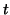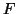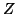Next: Acknowledgements Up: tr01cb1 Previous: -tests

# Conclusion

In this paper we have shown that it is possible to efficiently test, using only first-level parameter and (co-)variance estimates, general hypotheses for a mixed-effects2 group analysis model within the framework of the multi-level GLM under the BLUE for known variances. In particular, we have demonstrated the equivalence between a multi- level GLM and a single-level version if (and only if) the (co-)variance structure is modified appropriately. The result has a natural interpretation in that the variance used in the second-level analysis must be the sum of the (co-)variances from the individual and group levels. This eqivalence allows the second-level analysis to be carried out efficiently, only using first-level results, without any need to revisit the FMRI time-series data.

A further consequence of the model equivalence is that we can switch freely between the two forms of the model, depending on which one is more convenient at any one time. Also, the equivalence relation naturally extends to multi-level GLMs, so this framework encompasses more complex analysis scenarios, e.g. where data is grouped first across sessions and later across subjects.

This paper deliberately restricts itself to the issues involved in modelling and does not directly address the problem of estimation. The main problem in estimation for group studies in FMRI is the generally small number of subjects/sessions and the associated difficulty in obtaining accurate (co-)variance estimates. This problem will occur irrespective of the specific model used. Naturally, any advanced estimation technique can be used for this multi-level model. These problems of estimation have been investigated separately using a fully Bayesian approach . This is implemented within the current release version of FSL  and will be the topic of a forthcoming paper.

We have provided examples of how various group designs can be formulated within this general framework, including commonly used designs such as paired and unpaired-tests or-tests at the group level. The explicit equations in each of these cases can be derived easily from equations 7 and 10, such that the differences in the exact formulation of these tests and their assumptions become explicit. For example, all results in section 8 have been derived without the need for balanced designs, i.e. they allow for different first-level regressors. Balanced designs, therefore, are not a requirement of the multi-level GLM but potentially are a constraint introduced by a specific estimation technique. This becomes relevant when the first-level regressors need to be subject specific, e.g. are related to the subject's performance in a cognitive task.

To demonstrate some of the benefits of more flexible mixed-effects modelling some numerical simulations have been generated which contrasted the heteroscedastic model versus the standard homoscedastic (OLS) random-effects model. Using typical first-level variance structures from real FMRI data, these simulations demonstrate that by taking into account the relevant lower-level variances and heterogeneity amongst them, a substantial increase in higher-level-scores is possible.Next: Acknowledgements Up: tr01cb1 Previous: -tests
Christian Beckmann 2003-07-16